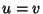## Morphism

A map between two objects in an abstract Category.

1. A general morphism is called a Homomorphism,

2. A morphismin a Category is a Monomorphism if, for any two morphisms,implies that,

3. A morphismin a Category is an Epimorphism if, for any two morphisms,implies,

4. A bijective morphism is called an Isomorphism (if there is an isomorphism between two objects, then we say they are isomorphic),

5. A surjective morphism from an object to itself is called an Endomorphism, and

6. An Isomorphism between an object and itself is called an Automorphism.